Anúncio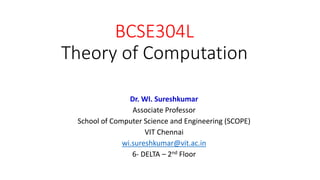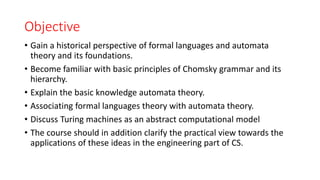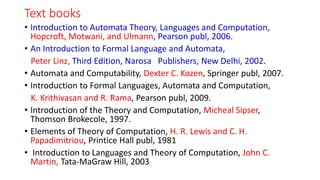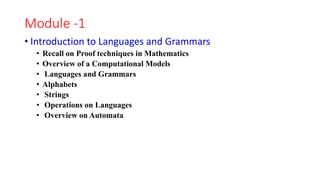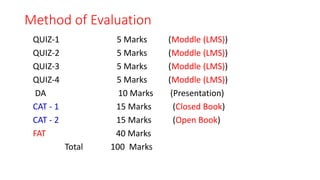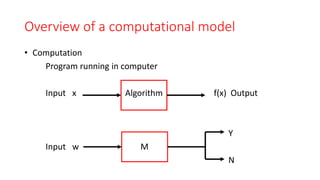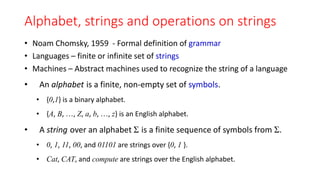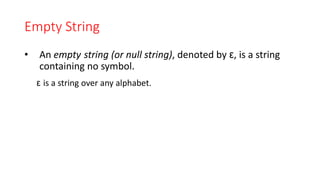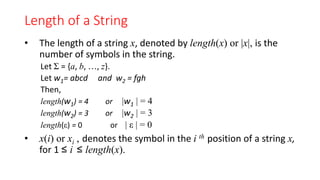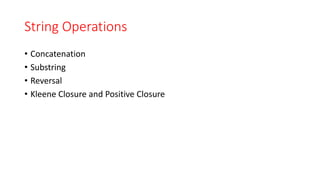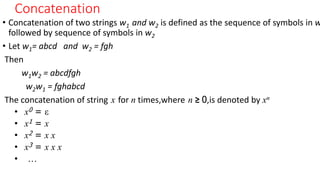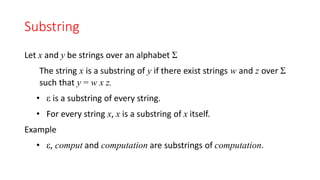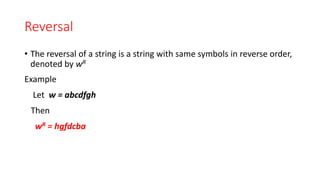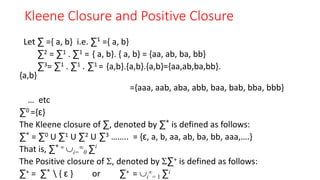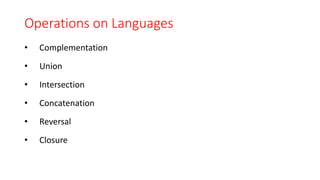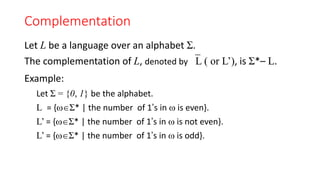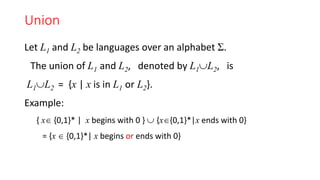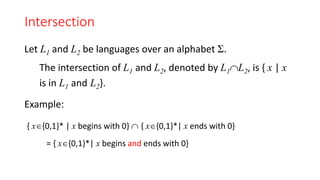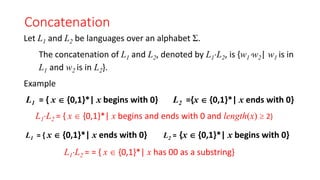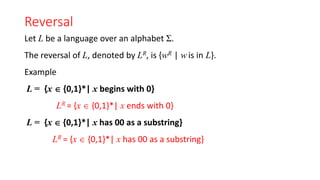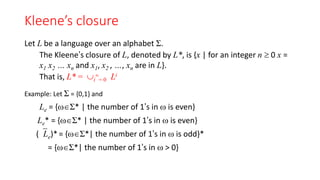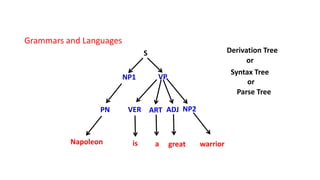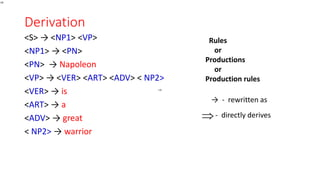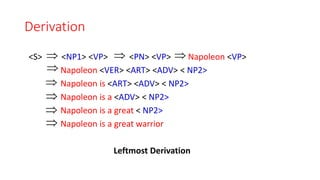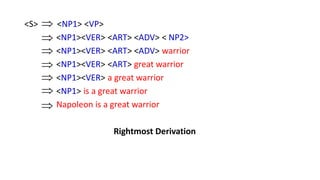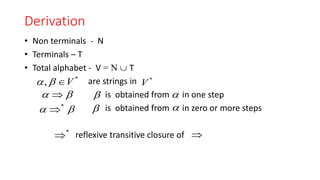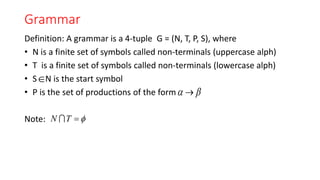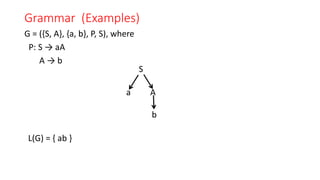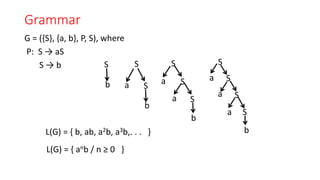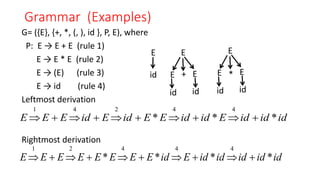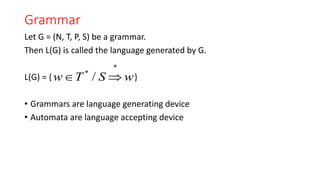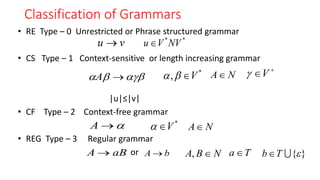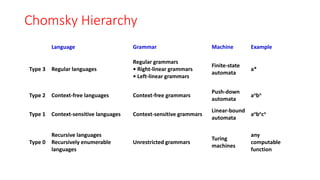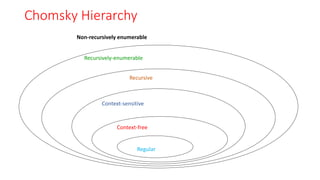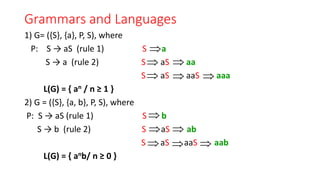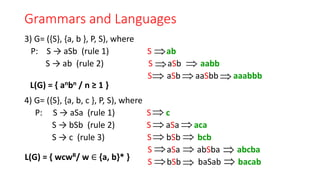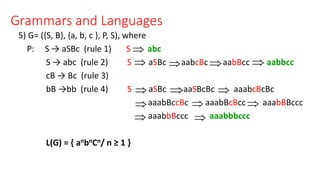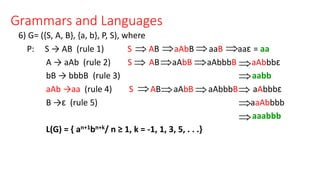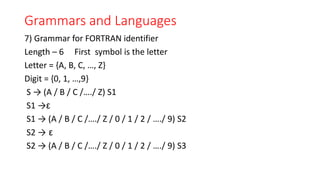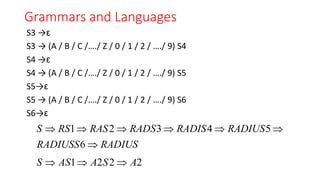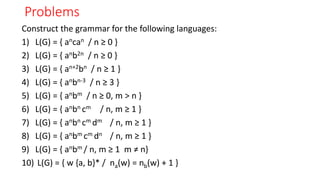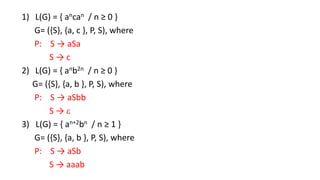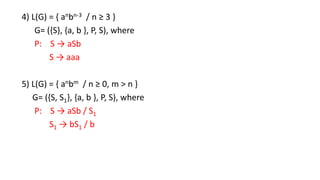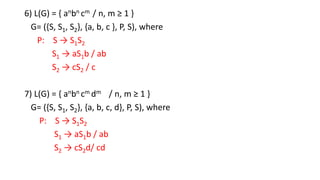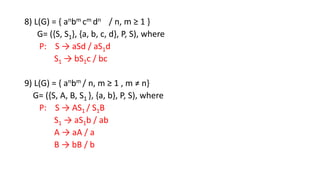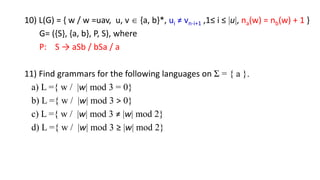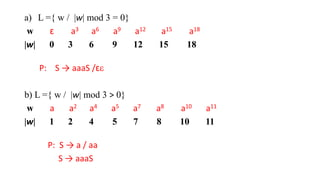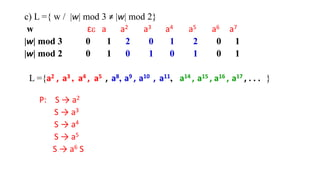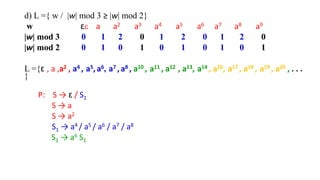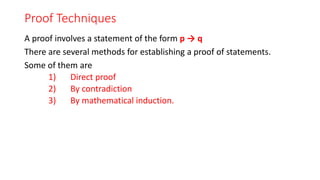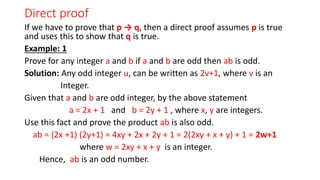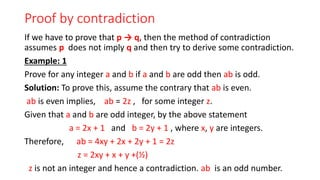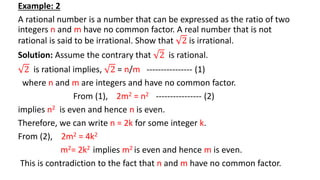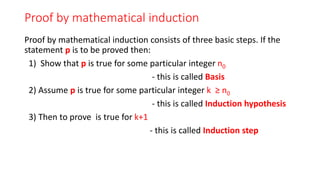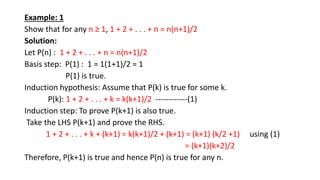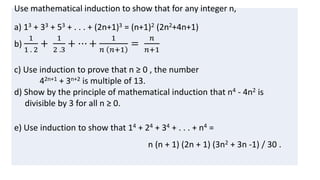1 de 70
Anúncio

### Module 1 TOC.pptx

1. BCSE304L Theory of Computation Dr. WI. Sureshkumar Associate Professor School of Computer Science and Engineering (SCOPE) VIT Chennai wi.sureshkumar@vit.ac.in 6- DELTA – 2nd Floor
2. Objective • Gain a historical perspective of formal languages and automata theory and its foundations. • Become familiar with basic principles of Chomsky grammar and its hierarchy. • Explain the basic knowledge automata theory. • Associating formal languages theory with automata theory. • Discuss Turing machines as an abstract computational model • The course should in addition clarify the practical view towards the applications of these ideas in the engineering part of CS.
3. Text books • Introduction to Automata Theory, Languages and Computation, Hopcroft, Motwani, and Ulmann, Pearson publ, 2006. • An Introduction to Formal Language and Automata, Peter Linz, Third Edition, Narosa Publishers, New Delhi, 2002. • Automata and Computability, Dexter C. Kozen, Springer publ, 2007. • Introduction to Formal Languages, Automata and Computation, K. Krithivasan and R. Rama, Pearson publ, 2009. • Introduction of the Theory and Computation, Micheal Sipser, Thomson Brokecole, 1997. • Elements of Theory of Computation, H. R. Lewis and C. H. Papadimitriou, Printice Hall publ, 1981 • Introduction to Languages and Theory of Computation, John C. Martin, Tata-MaGraw Hill, 2003
4. Module -1 • Introduction to Languages and Grammars • Recall on Proof techniques in Mathematics • Overview of a Computational Models • Languages and Grammars • Alphabets • Strings • Operations on Languages • Overview on Automata
5. Method of Evaluation QUIZ-1 5 Marks (Moddle (LMS)) QUIZ-2 5 Marks (Moddle (LMS)) QUIZ-3 5 Marks (Moddle (LMS)) QUIZ-4 5 Marks (Moddle (LMS)) DA 10 Marks (Presentation) CAT - 1 15 Marks (Closed Book) CAT - 2 15 Marks (Open Book) FAT 40 Marks Total 100 Marks
6. Overview of a computational model • Computation Program running in computer Input x Algorithm f(x) Output Y Input w M N
7. Alphabet, strings and operations on strings • Noam Chomsky, 1959 - Formal definition of grammar • Languages – finite or infinite set of strings • Machines – Abstract machines used to recognize the string of a language • An alphabet is a finite, non-empty set of symbols. • {0,1} is a binary alphabet. • {A, B, …, Z, a, b, …, z} is an English alphabet. • A string over an alphabet Σ is a finite sequence of symbols from Σ. • 0, 1, 11, 00, and 01101 are strings over {0, 1 }. • Cat, CAT, and compute are strings over the English alphabet.
8. Empty String • An empty string (or null string), denoted by ε, is a string containing no symbol. ε is a string over any alphabet.
9. Length of a String • The length of a string x, denoted by length(x) or |x|, is the number of symbols in the string. Let Σ = {a, b, …, z}. Let w1= abcd and w2 = fgh Then, length(w1) = 4 or |w1 | = 4 length(w2) = 3 or |w2 | = 3 length(ε) = 0 or | ε | = 0 • x(i) or xi , denotes the symbol in the i th position of a string x, for 1 ≤ i ≤ length(x).
10. String Operations • Concatenation • Substring • Reversal • Kleene Closure and Positive Closure
11. Concatenation • Concatenation of two strings w1 and w2 is defined as the sequence of symbols in w followed by sequence of symbols in w2 • Let w1= abcd and w2 = fgh Then w1w2 = abcdfgh w2w1 = fghabcd The concatenation of string x for n times,where n ≥ 0,is denoted by xn • x0 = ε • x1 = x • x2 = x x • x3 = x x x • …
12. Substring Let x and y be strings over an alphabet Σ The string x is a substring of y if there exist strings w and z over Σ such that y = w x z. • ε is a substring of every string. • For every string x, x is a substring of x itself. Example • ε, comput and computation are substrings of computation.
13. Reversal • The reversal of a string is a string with same symbols in reverse order, denoted by wR Example Let w = abcdfgh Then wR = hgfdcba
14. Kleene Closure and Positive Closure Let ∑ ={ a, b} i.e. ∑1 ={ a, b} ∑2 = ∑1 . ∑1 = { a, b}. { a, b} = {aa, ab, ba, bb} ∑3= ∑1 . ∑1 . ∑1 = {a,b}.{a,b}.{a,b}={aa,ab,ba,bb}. {a,b} ={aaa, aab, aba, abb, baa, bab, bba, bbb} … etc ∑0 ={ε} The Kleene closure of ∑, denoted by ∑* is defined as follows: ∑* = ∑0 U ∑1 U ∑2 U ∑3 …….. = {ε, a, b, aa, ab, ba, bb, aaa,….} That is, ∑* = i=  0 ∑i The Positive closure of , denoted by ∑+ is defined as follows: ∑+ = ∑* { ε } or ∑+ = i  = 1 ∑i
15. Operations on Languages • Complementation • Union • Intersection • Concatenation • Reversal • Closure
16. Complementation Let L be a language over an alphabet Σ. The complementation of L, denoted by L ( or L’), is Σ*– L. Example: Let Σ = {0, 1} be the alphabet. L = {Σ* | the number of 1’s in  is even}. L’ = {Σ* | the number of 1’s in  is not even}. L’ = {Σ* | the number of 1’s in  is odd}.
17. Union Let L1 and L2 be languages over an alphabet Σ. The union of L1 and L2, denoted by L1L2, is L1L2 = {x | x is in L1 or L2}. Example: { x {0,1}* | x begins with 0 }  {x{0,1}*|x ends with 0} = {x  {0,1}*| x begins or ends with 0}
18. Intersection Let L1 and L2 be languages over an alphabet Σ. The intersection of L1 and L2, denoted by L1L2, is { x | x is in L1 and L2}. Example: { x{0,1}* | x begins with 0}  { x{0,1}*| x ends with 0} = { x{0,1}*| x begins and ends with 0}
19. Concatenation Let L1 and L2 be languages over an alphabet Σ. The concatenation of L1 and L2, denoted by L1L2, is {w1w2| w1 is in L1 and w2 is in L2}. Example L1 = { x  {0,1}*| x begins with 0} L2 ={x  {0,1}*| x ends with 0} L1L2 = { x  {0,1}*| x begins and ends with 0 and length(x)  2} L1 = { x  {0,1}*| x ends with 0} L2 = {x  {0,1}*| x begins with 0} L1L2 = = { x  {0,1}*| x has 00 as a substring}
20. Reversal Let L be a language over an alphabet Σ. The reversal of L, denoted by LR, is {wR | w is in L}. Example L = {x  {0,1}*| x begins with 0} LR = {x  {0,1}*| x ends with 0} L = {x  {0,1}*| x has 00 as a substring} LR = {x  {0,1}*| x has 00 as a substring}
21. Kleene’s closure Let L be a language over an alphabet Σ. The Kleene’s closure of L, denoted by L*, is {x | for an integer n  0 x = x1 x2 … xn and x1, x2 , …, xn are in L}. That is, L* = i  = 0 Li Example: Let Σ = {0,1} and Le = {Σ* | the number of 1’s in  is even} Le* = {Σ* | the number of 1’s in  is even} (Le)*= {Σ*| the number of 1’s in  is odd}* = {Σ*| the number of 1’s in  > 0}
22. Grammars and Languages Napoleon S NP1 VP PN VER ART ADJ NP2 is a great warrior Derivation Tree Syntax Tree or or Parse Tree
23. Derivation <S> → <NP1> <VP> <NP1> → <PN> <PN> → Napoleon <VP> → <VER> <ART> <ADV> < NP2> <VER> → is <ART> → a <ADV> → great < NP2> → warrior Rules or Productions or Production rules → - rewritten as - directly derives  
24. Derivation <S> <NP1> <VP> <PN> <VP> Napoleon <VP> Napoleon <VER> <ART> <ADV> < NP2> Napoleon is <ART> <ADV> < NP2> Napoleon is a <ADV> < NP2> Napoleon is a great < NP2> Napoleon is a great warrior Leftmost Derivation        
25. <S> <NP1> <VP> <NP1><VER> <ART> <ADV> < NP2> <NP1><VER> <ART> <ADV> warrior <NP1><VER> <ART> great warrior <NP1><VER> a great warrior <NP1> is a great warrior Napoleon is a great warrior Rightmost Derivation       
26. Derivation • Non terminals - N • Terminals – T • Total alphabet - V = N  T are strings in is obtained from in one step is obtained from in zero or more steps reflexive transitive closure of * , V    * V        *    *  
27. Grammar Definition: A grammar is a 4-tuple G = (N, T, P, S), where • N is a finite set of symbols called non-terminals (uppercase alph) • T is a finite set of symbols called non-terminals (lowercase alph) • S N is the start symbol • P is the set of productions of the form Note:       T N 
28. Grammar (Examples) G = ({S, A}, {a, b}, P, S), where P: S → aA A → b S a A b L(G) = { ab }
29. Grammar G = ({S}, {a, b}, P, S), where P: S → aS S → b S b S a S b S a S b a S S a S b a S a S L(G) = { b, ab, a2b, a3b,. . . } L(G) = { anb / n ≥ 0 }
30. Grammar (Examples) id id id E id id E E id E id E E E * * * 4 4 2 4 1           E id G= ({E}, {+, *, (, ), id }, P, E), where P: E → E + E (rule 1) E → E * E (rule 2) E → (E) (rule 3) E → id (rule 4) Leftmost derivation Rightmost derivation id E E E id * id id id id id E id E E E E E E E E * * * * 4 4 4 2 1           + id E E E id
31. Grammar Let G = (N, T, P, S) be a grammar. Then L(G) is called the language generated by G. L(G) = { } • Grammars are language generating device • Automata are language accepting device w S T w * * /  
32. Classification of Grammars • RE Type – 0 Unrestricted or Phrase structured grammar • CS Type – 1 Context-sensitive or length increasing grammar |u|≤|v| • CF Type – 2 Context-free grammar • REG Type – 3 Regular grammar or v u  * * NV V u     A * , V    N A  V    A * V   N A aB A  b A  N B A  , T a } {  T b
33. Chomsky Hierarchy Language Grammar Machine Example Type 3 Regular languages Regular grammars • Right-linear grammars • Left-linear grammars Finite-state automata a* Type 2 Context-free languages Context-free grammars Push-down automata anbn Type 1 Context-sensitive languages Context-sensitive grammars Linear-bound automata anbncn Type 0 Recursive languages Recursively enumerable languages Unrestricted grammars Turing machines any computable function
34. Chomsky Hierarchy Non-recursively enumerable Recursively-enumerable Recursive Context-sensitive Context-free Regular
35. Grammars and Languages 1) G= ({S}, {a}, P, S), where P: S → aS (rule 1) S a S → a (rule 2) S aS aa S aS aaS aaa L(G) = { an / n ≥ 1 } 2) G = ({S}, {a, b}, P, S), where P: S → aS (rule 1) S b S → b (rule 2) S aS ab S aS aaS aab L(G) = { anb/ n ≥ 0 }            
36. Grammars and Languages 3) G= ({S}, {a, b }, P, S), where P: S → aSb (rule 1) S ab S → ab (rule 2) S aSb aabb S aSb aaSbb aaabbb 4) G= ({S}, {a, b, c }, P, S), where P: S → aSa (rule 1) S c S → bSb (rule 2) S aSa aca S → c (rule 3) S bSb bcb S aSa abSba abcba S bSb baSab bacab L(G) = { anbn / n ≥ 1 } L(G) = { wcwR/ w ∈ {a, b}* }                 
37. Grammars and Languages 5) G= ({S, B}, {a, b, c }, P, S), where P: S → aSBc (rule 1) S abc S → abc (rule 2) S aSBc aabcBc aabBcc aabbcc cB → Bc (rule 3) bB →bb (rule 4) S aSBc aaSBcBc aaabcBcBc aaabBccBc aaabBcBcc aaabBBccc aaabbBccc aaabbbccc L(G) = { anbnCn/ n ≥ 1 }             
38. Grammars and Languages 6) G= ({S, A, B}, {a, b}, P, S), where P: S → AB (rule 1) S AB aAbB aaB aaε = aa A → aAb (rule 2) S AB aAbB aAbbbB aAbbbε bB → bbbB (rule 3) aabb aAb →aa (rule 4) S AB aAbB aAbbbB aAbbbε B →ε (rule 5) aaAbbbb aaabbb L(G) = { an+1bn+k/ n ≥ 1, k = -1, 1, 3, 5, . . .}               
39. Grammars and Languages 7) Grammar for FORTRAN identifier Length – 6 First symbol is the letter Letter = {A, B, C, …, Z} Digit = {0, 1, …,9} S → (A / B / C /…./ Z) S1 S1 →ε S1 → (A / B / C /…./ Z / 0 / 1 / 2 / …./ 9) S2 S2 → ε S2 → (A / B / C /…./ Z / 0 / 1 / 2 / …./ 9) S3
40. Grammars and Languages S3 →ε S3 → (A / B / C /…./ Z / 0 / 1 / 2 / …./ 9) S4 S4 →ε S4 → (A / B / C /…./ Z / 0 / 1 / 2 / …./ 9) S5 S5→ε S5 → (A / B / C /…./ Z / 0 / 1 / 2 / …./ 9) S6 S6→ε RADIUS RADIUSS RADIUS RADIS RADS RAS RS S        6 5 4 3 2 1 2 2 2 1 A S A AS S   
41. Problems Construct the grammar for the following languages: 1) L(G) = { ancan / n ≥ 0 } 2) L(G) = { anb2n / n ≥ 0 } 3) L(G) = { an+2bn / n ≥ 1 } 4) L(G) = { anbn-3 / n ≥ 3 } 5) L(G) = { anbm / n ≥ 0, m ˃ n } 6) L(G) = { anbn cm / n, m ≥ 1 } 7) L(G) = { anbn cm dm / n, m ≥ 1 } 8) L(G) = { anbm cm dn / n, m ≥ 1 } 9) L(G) = { anbm / n, m ≥ 1 m ≠ n} 10) L(G) = { w {a, b}* / na(w) = nb(w) + 1 }
42. 1) L(G) = { ancan / n ≥ 0 } G= ({S}, {a, c }, P, S), where P: S → aSa S → c 2) L(G) = { anb2n / n ≥ 0 } G= ({S}, {a, b }, P, S), where P: S → aSbb S →  3) L(G) = { an+2bn / n ≥ 1 } G= ({S}, {a, b }, P, S), where P: S → aSb S → aaab
43. 4) L(G) = { anbn-3 / n ≥ 3 } G= ({S}, {a, b }, P, S), where P: S → aSb S → aaa 5) L(G) = { anbm / n ≥ 0, m ˃ n } G= ({S, S1}, {a, b }, P, S), where P: S → aSb / S1 S1 → bS1 / b
44. 6) L(G) = { anbn cm / n, m ≥ 1 } G= ({S, S1, S2}, {a, b, c }, P, S), where P: S → S1S2 S1 → aS1b / ab S2 → cS2 / c 7) L(G) = { anbn cm dm / n, m ≥ 1 } G= ({S, S1, S2}, {a, b, c, d}, P, S), where P: S → S1S2 S1 → aS1b / ab S2 → cS2d/ cd
45. 8) L(G) = { anbm cm dn / n, m ≥ 1 } G= ({S, S1}, {a, b, c, d}, P, S), where P: S → aSd / aS1d S1 → bS1c / bc 9) L(G) = { anbm / n, m ≥ 1 , m ≠ n} G= ({S, A, B, S1 }, {a, b}, P, S), where P: S → AS1 / S1B S1 → aS1b / ab A → aA / a B → bB / b
46. 10) L(G) = { w / w =uav, u, v  {a, b}*, ui ≠ vn-i+1 ,1≤ i ≤ |u|, na(w) = nb(w) + 1 } G= ({S}, {a, b}, P, S), where P: S → aSb / bSa / a 11) Find grammars for the following languages on Σ = { a }. a) L ={ w / |w| mod 3 = 0} b) L ={ w / |w| mod 3 ˃ 0} c) L ={ w / |w| mod 3 ≠ |w| mod 2} d) L ={ w / |w| mod 3 ≥ |w| mod 2}
47. a) L ={ w / |w| mod 3 = 0} w ε a3 a6 a9 a12 a15 a18 |w| 0 3 6 9 12 15 18 P: S → aaaS /ε b) L ={ w / |w| mod 3 ˃ 0} w a a2 a4 a5 a7 a8 a10 a11 |w| 1 2 4 5 7 8 10 11 P: S → a / aa S → aaaS
48. c) L ={ w / |w| mod 3 ≠ |w| mod 2} w ε a a2 a3 a4 a5 a6 a7 |w| mod 3 0 1 2 0 1 2 0 1 |w| mod 2 0 1 0 1 0 1 0 1 L ={a2 , a3 , a4 , a5 , a8, a9 , a10 , a11, a14 , a15 , a16 , a17 , . . . } P: S → a2 S → a3 S → a4 S → a5 S → a6 S
49. d) L ={ w / |w| mod 3 ≥ |w| mod 2} w ε a a2 a3 a4 a5 a6 a7 a8 a9 |w| mod 3 0 1 2 0 1 2 0 1 2 0 |w| mod 2 0 1 0 1 0 1 0 1 0 1 L ={ε , a ,a2 , a4 , a5, a6, a7 , a8 , a10 , a11 , a12 , a13, a14 , a16, a17 , a18 , a19 , a20 , . . . } P: S → ε / S1 S → a S → a2 S1 → a4 / a5 / a6 / a7 / a8 S1 → a6 S1
50. Proof Techniques A proof involves a statement of the form p → q There are several methods for establishing a proof of statements. Some of them are 1) Direct proof 2) By contradiction 3) By mathematical induction.
51. Direct proof If we have to prove that p → q, then a direct proof assumes p is true and uses this to show that q is true. Example: 1 Prove for any integer a and b if a and b are odd then ab is odd. Solution: Any odd integer u, can be written as 2v+1, where v is an Integer. Given that a and b are odd integer, by the above statement a = 2x + 1 and b = 2y + 1 , where x, y are integers. Use this fact and prove the product ab is also odd. ab = (2x +1) (2y+1) = 4xy + 2x + 2y + 1 = 2(2xy + x + y) + 1 = 2w+1 where w = 2xy + x + y is an integer. Hence, ab is an odd number.
52. Proof by contradiction If we have to prove that p → q, then the method of contradiction assumes p does not imply q and then try to derive some contradiction. Example: 1 Prove for any integer a and b if a and b are odd then ab is odd. Solution: To prove this, assume the contrary that ab is even. ab is even implies, ab = 2z , for some integer z. Given that a and b are odd integer, by the above statement a = 2x + 1 and b = 2y + 1 , where x, y are integers. Therefore, ab = 4xy + 2x + 2y + 1 = 2z z = 2xy + x + y +(½) z is not an integer and hence a contradiction. ab is an odd number.
53. Example: 2 A rational number is a number that can be expressed as the ratio of two integers n and m have no common factor. A real number that is not rational is said to be irrational. Show that 2 is irrational. Solution: Assume the contrary that 2 is rational. 2 is rational implies, 2 = n/m ---------------- (1) where n and m are integers and have no common factor. From (1), 2m2 = n2 ---------------- (2) implies n2 is even and hence n is even. Therefore, we can write n = 2k for some integer k. From (2), 2m2 = 4k2 m2= 2k2 implies m2 is even and hence m is even. This is contradiction to the fact that n and m have no common factor.
54. Proof by mathematical induction Proof by mathematical induction consists of three basic steps. If the statement p is to be proved then: 1) Show that p is true for some particular integer n0 - this is called Basis 2) Assume p is true for some particular integer k ≥ n0 - this is called Induction hypothesis 3) Then to prove is true for k+1 - this is called Induction step
55. Example: 1 Show that for any n ≥ 1, 1 + 2 + . . . + n = n(n+1)/2 Solution: Let P(n) : 1 + 2 + . . . + n = n(n+1)/2 Basis step: P(1) : 1 = 1(1+1)/2 = 1 P(1) is true. Induction hypothesis: Assume that P(k) is true for some k. P(k): 1 + 2 + . . . + k = k(k+1)/2 ------------(1) Induction step: To prove P(k+1) is also true. Take the LHS P(k+1) and prove the RHS. 1 + 2 + . . . + k + (k+1) = k(k+1)/2 + (k+1) = (k+1) (k/2 +1) using (1) = (k+1)(k+2)/2 Therefore, P(k+1) is true and hence P(n) is true for any n.
56. Use mathematical induction to show that for any integer n, a) 13 + 33 + 53 + . . . + (2n+1)3 = (n+1)2 (2n2+4n+1) b) 1 1 . 2 + 1 2 .3 + ⋯ + 1 𝑛 𝑛+1 = 𝑛 𝑛+1 c) Use induction to prove that n ≥ 0 , the number 42n+1 + 3n+2 is multiple of 13. d) Show by the principle of mathematical induction that n4 - 4n2 is divisible by 3 for all n ≥ 0. e) Use induction to show that 14 + 24 + 34 + . . . + n4 = n (n + 1) (2n + 1) (3n2 + 3n -1) / 30 .
Anúncio